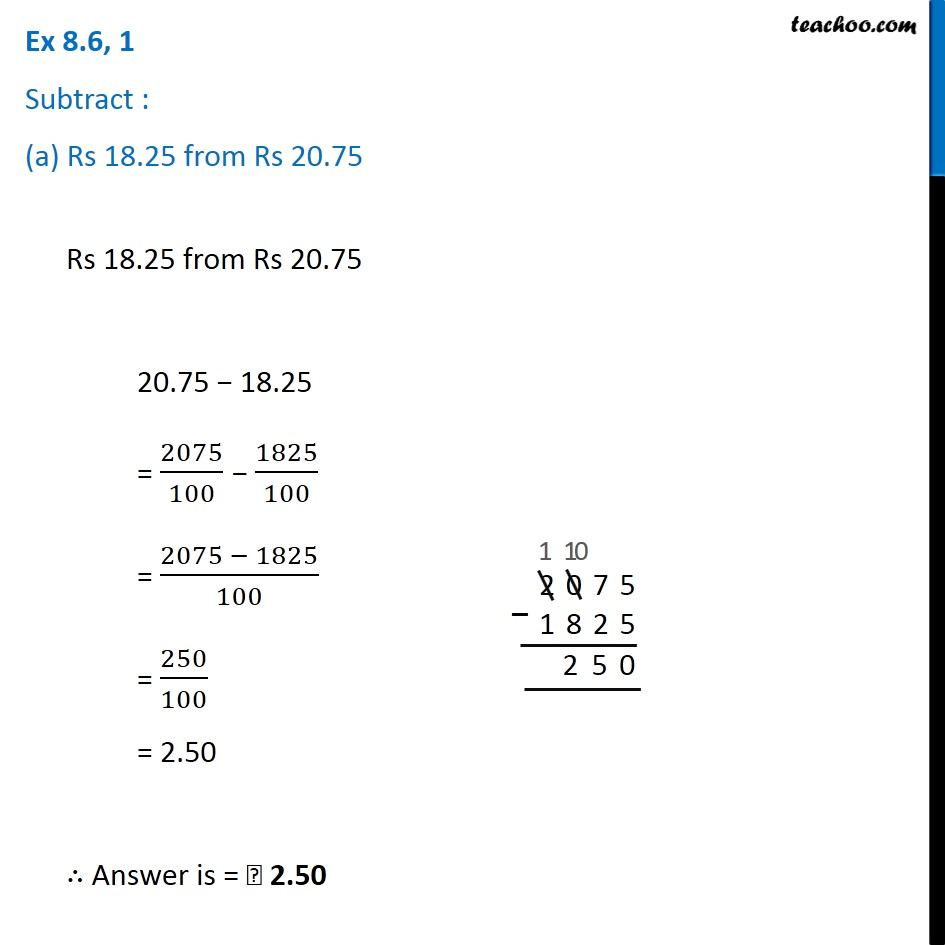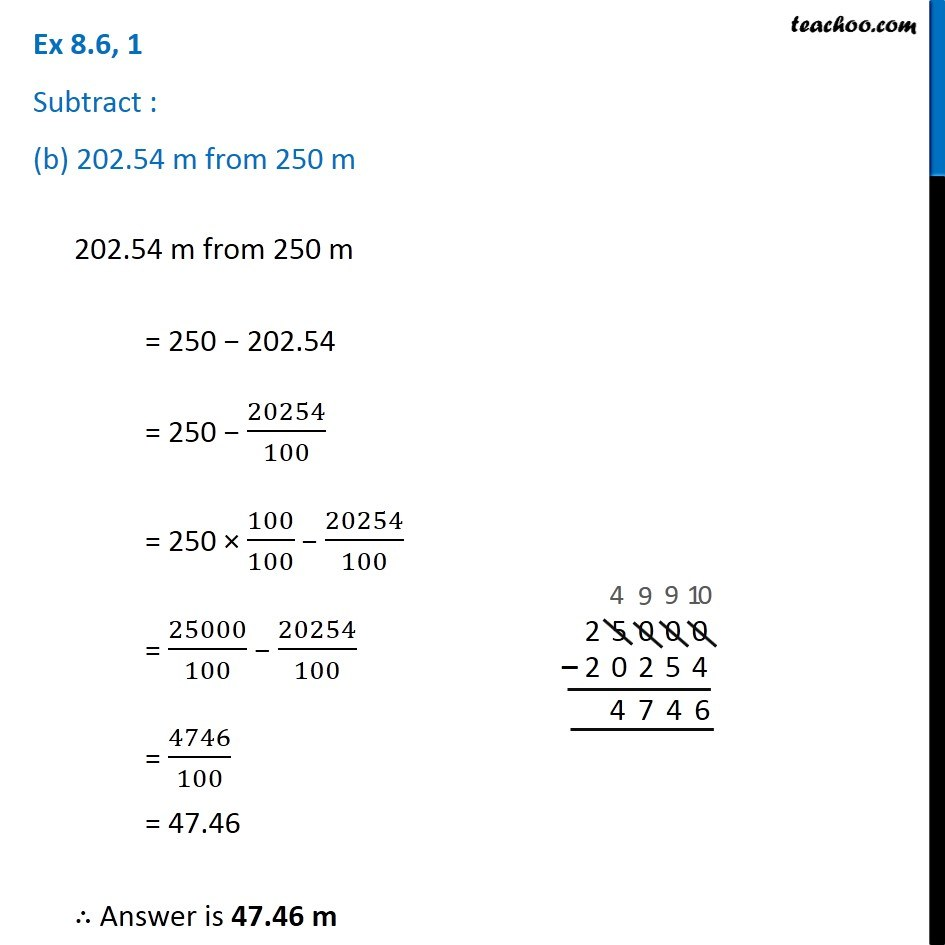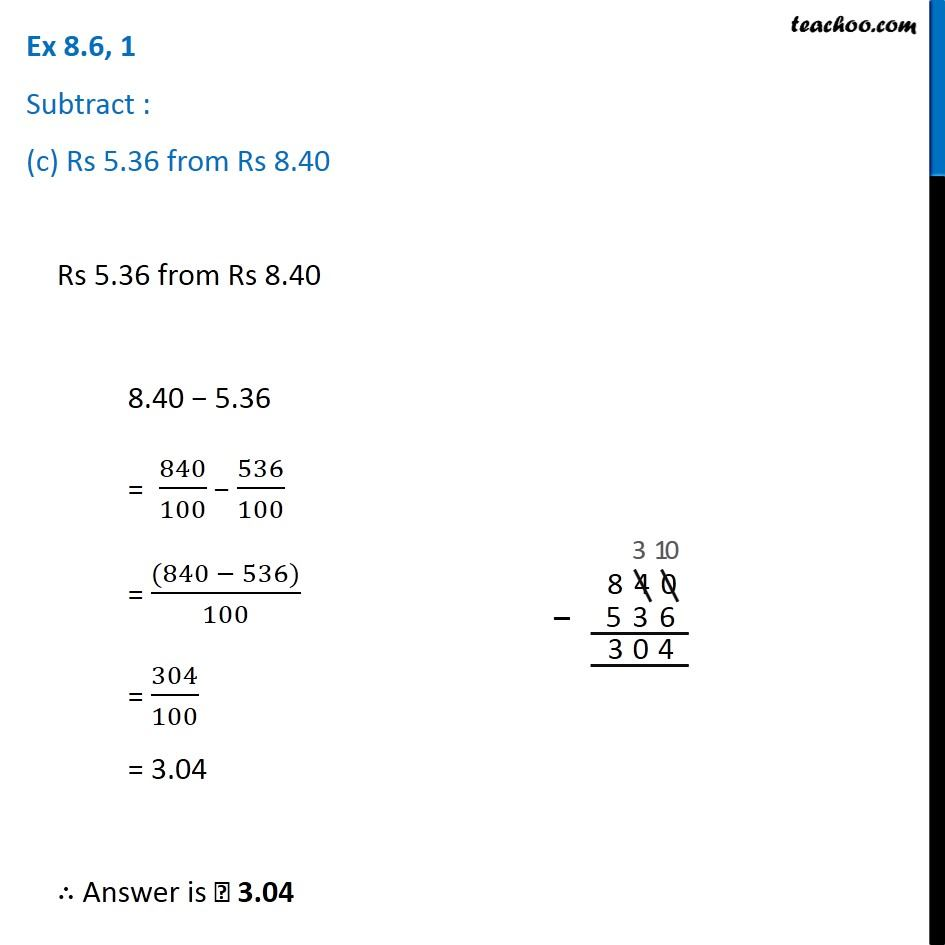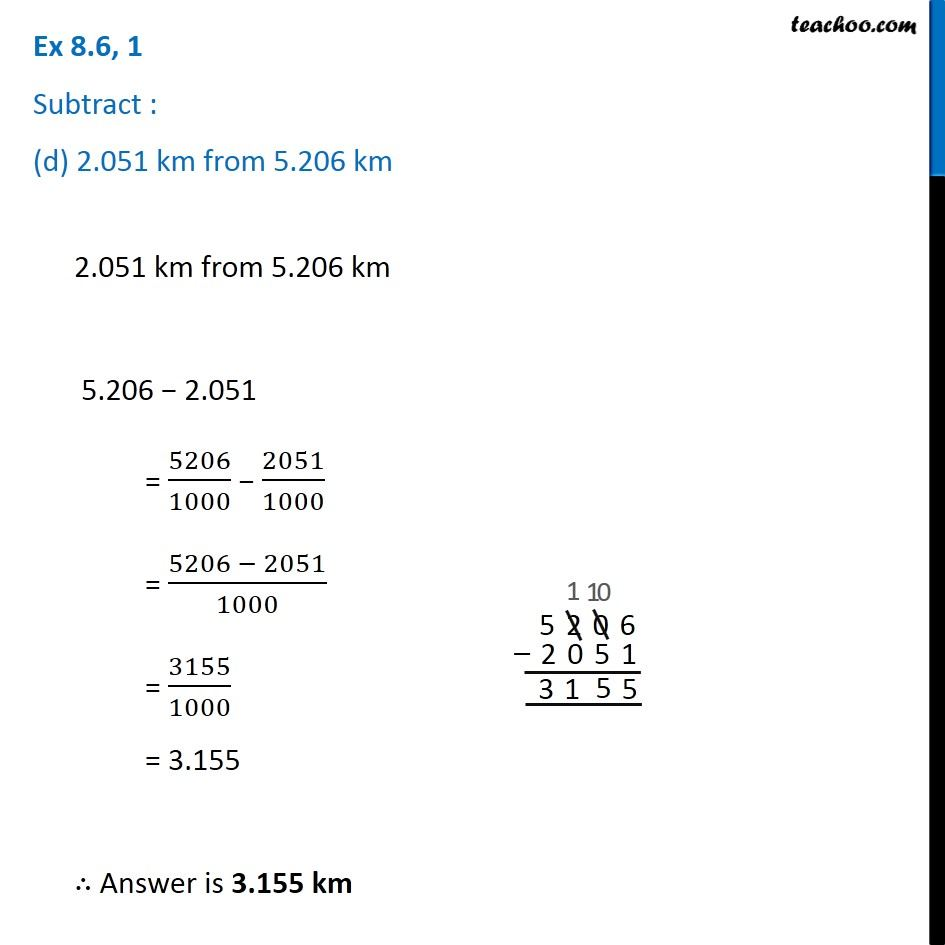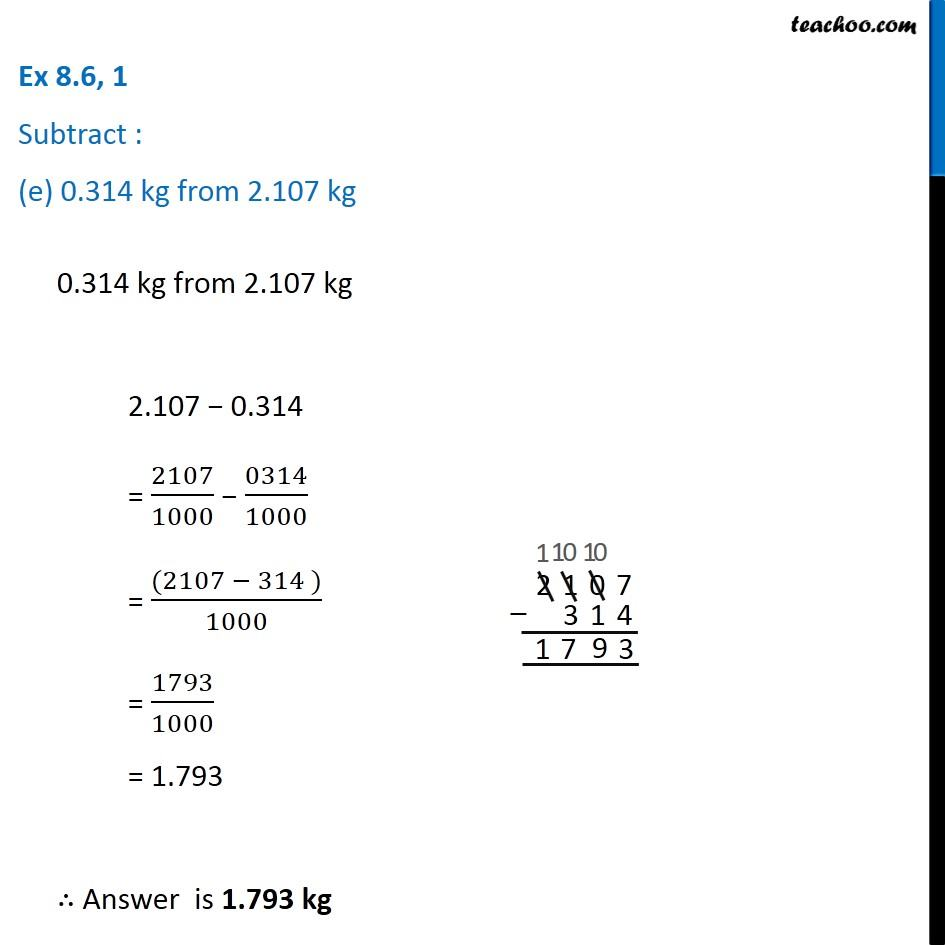Subscribe to our Youtube Channel - https://you.tube/teachoo

1. Chapter 8 Class 6 Decimals
2. Concept wise
3. Subtraction of decimal numbers

Transcript

Ex 8.6, 1 Subtract : (a) Rs 18.25 from Rs 20.75Rs 18.25 from Rs 20.75 20.75 − 18.25 = 2075/100 − 1825/100 = (2075 − 1825)/100 = 250/100 = 2.50 ∴ Answer is = ₹ 2.50 Ex 8.6, 1 Subtract : (b) 202.54 m from 250 m202.54 m from 250 m = 250 − 202.54 = 250 − 20254/100 = 250 × 100/100 − 20254/100 = 25000/100 − 20254/100 = 4746/100 = 47.46 ∴ Answer is 47.46 m Ex 8.6, 1 Subtract : (c) Rs 5.36 from Rs 8.40 Rs 5.36 from Rs 8.40 8.40 − 5.36 = 840/100 − 536/100 = ((840 − 536))/100 = 304/100 = 3.04 ∴ Answer is ₹ 3.04 Ex 8.6, 1 Subtract : (d) 2.051 km from 5.206 km2.051 km from 5.206 km 5.206 − 2.051 = 5206/1000 − 2051/1000 = (5206 − 2051)/1000 = 3155/1000 = 3.155 ∴ Answer is 3.155 km Ex 8.6, 1 Subtract : (e) 0.314 kg from 2.107 kg0.314 kg from 2.107 kg 2.107 − 0.314 = 2107/1000 − 0314/1000 = ((2107 − 314 ))/1000 = 1793/1000 = 1.793 ∴ Answer is 1.793 kg

Subtraction of decimal numbers

About the AuthorDavneet Singh
Davneet Singh is a graduate from Indian Institute of Technology, Kanpur. He has been teaching from the past 9 years. He provides courses for Maths and Science at Teachoo.